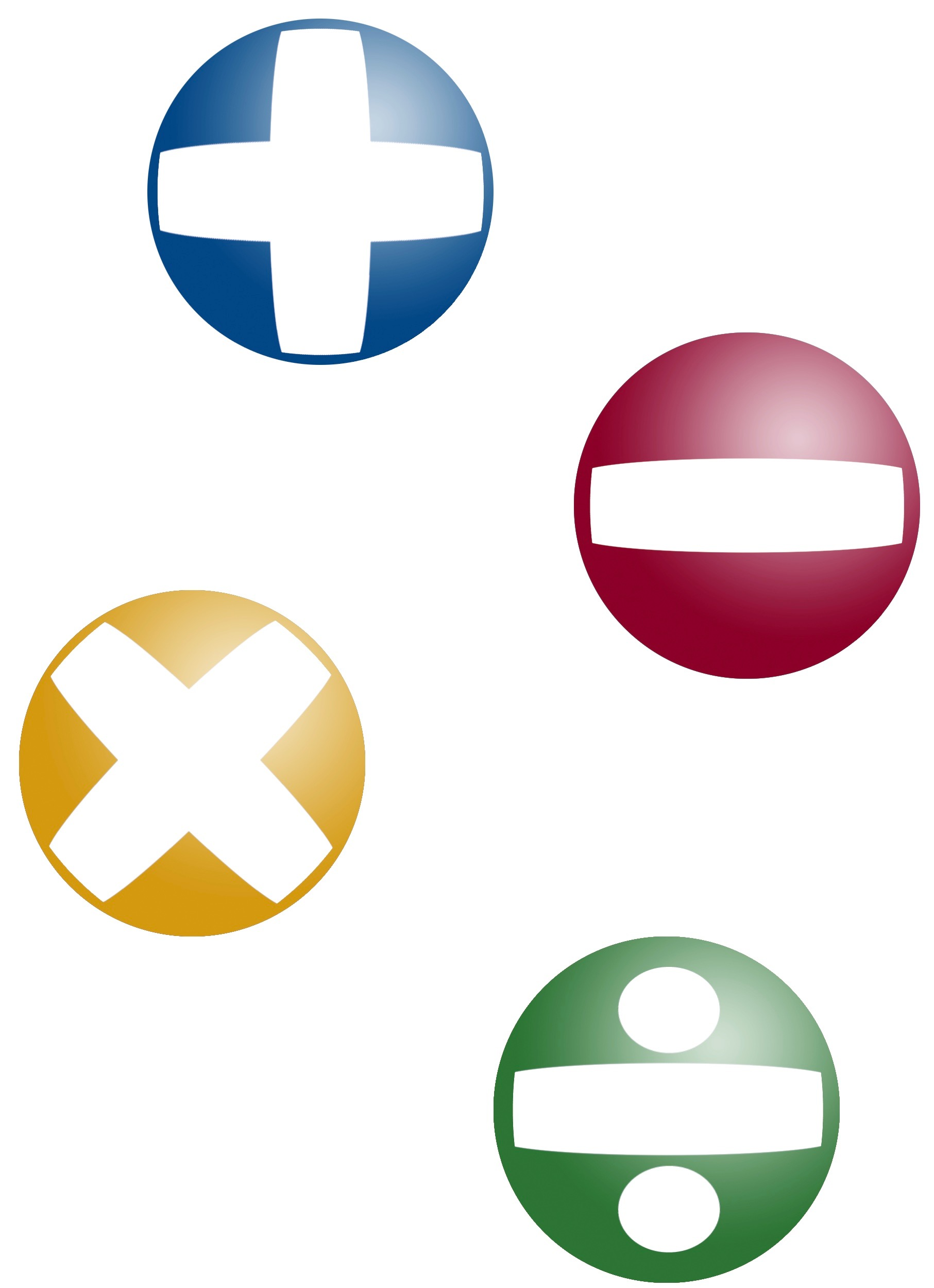## ________________

The team enjoys playing with these digit combination challenges posed by the coach.

## ________________### Making 25

Using only +, -, ×, ÷, exponents, decimal points, parentheses, and concatenation (that is, combining two digits into another number; for instance, putting 1 and 2 together to make 21 or 12), find two ways to make 25 using 1, 4, and 6. No roots, factorials, repeating decimals, or other math functions are permitted.

### Two Ways to 32

Now try making 32 two ways: first using 4, 5, and 7, then using 5, 6, and 7.

## Solutions to week 109

In Commuters’ Dilemma, the equilibrium driving time is 140 minutes. In Construction Conundrum, the equilibrium driving time is 130 minutes.

Commuters’ Dilemma answer explained: There are four paths a commuter can consider: HAS, HABS, HBS and HBAS. The HBAS path takes a constant time of 160 min and will never be chosen because it will always take longer than the others. Suppose J cars take path HAS, K cars take path HABS and L cars take path HBS, where J+K+L = 19,000. Then the commute times for these three paths are:

tJ = (J+K)/200 +50
tK = (J+K)/200+10+(K+L)/100
tL = 100+(K+L)/100

In equilibrium, these three times will be the same, giving J = 15,000, K = 3,000 and L = 1,000
so that tJ = tK = tL = 140 min.

Construction Conundrum answer explained: During the week of construction suppose J cars take path HAS, K cars take path HABS and L cars take path HBS, where J+K+L = 19,000. Then the commute times for these three paths are:

tJ = (J+K)/200 +50
tK = (J+K)/200+20+(K+L)/100
tL = 100+(K+L)/100

In equilibrium, these three times will be the same, giving J = 16,000, K = 0 and L = 3,000
so that tJ = tK = tL = 130 min.

It’s a remarkable fact that the 10-minute extra delay on AB owing to construction decreases everyone’s commute time by 10 minutes. This is an example of what is known as Braess’s Paradox.

## Recent Weeks

Links to all of the puzzles and solutions are on the Complete Varsity Math page.

Come back next week for answers and more puzzles.